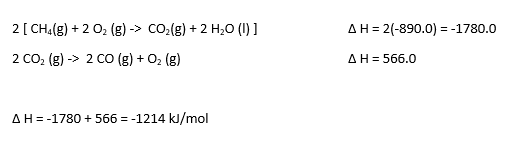# AP Chemistry : Thermodynamics

## Example Questions

### Example Question #94 : Thermochemistry And Kinetics

Suppose that a reaction with an equilibrium constant equal to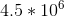occurs while at standard state conditions. Which of the following is true regarding this reaction?

The Gibbs free energy change for this reaction is positive and the reaction is nonspontaneous

The Gibbs free energy change for this reaction is negative and the reaction is spontaneous

The Gibbs free energy change for this reaction is negative and the reaction is nonspontaneous

The Gibbs free energy change for this reaction is positive and the reaction is spontaneous

The Gibbs free energy change for this reaction is negative and the reaction is spontaneous

Explanation:

For this question, we're told that a reaction is being run under standard conditions, and that the equilibrium constant for this reaction is much greater than 1. With this information in mind, we can find the correct answer without even having to resort to math. Since the equilibrium constant is greater than one, we know that the products of this reaction are favored over the reactants. And since the products are favored, this means that the reaction must be shifted to the right, in which case it is spontaneous. It's important to know that a spontaneous reaction will always have a negative change in Gibb's energy.

For completion's sake, however, we can show that the Gibb's free energy change is negative by utilizing the free energy change equation.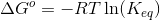From this equation, we can see that if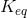is greater than one (as it is in the reaction for this question) the natural logarithm of this value will also be positive. And since the ideal gas constant and the absolute temperature are also positive values, the product of all these values will be positive. But, there is a negative sign in front of these terms, hence making our final answer negative.

### Example Question #95 : Thermochemistry And Kinetics

Which of the following situations describes a reaction that can never be spontaneous?

The entropy increases and the enthalpy decreases

The entropy decreases and the enthalpy decreases

The entropy increases and the enthalpy increases

The entropy decreases and the enthalpy increases

The entropy decreases and the enthalpy increases

Explanation:

In this question, we're being asked to provide a circumstance that will never result in a spontaneous reaction. In other words, which answer choice presents a situation that will always be non-spontaneous.

To evaluate the spontaneity of a reaction, it's essential to look at the change in the Gibb's free energy of that reaction. A negative change results in a spontaneous reaction, whereas a positive change results in a non-spontaneous reaction.

We can see that each of the answer choices mentions enthalpy and entropy. Therefore, we need to be able to relate these two terms with the Gibb's free energy term. To do this, we can make use of the following expression.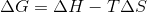Again, in order to have a non-spontaneous reaction, the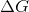term shown above needs to have a positive value.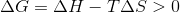From the above expression, we can conclude that any process that has a positive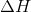and a negative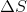will always be positive, and hence will always result in a non-spontaneous reaction.

### Example Question #96 : Thermochemistry And Kinetics

A chemical reaction has the following changes in enthalpy and entropy.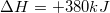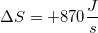What is the temperature range for this reaction that allow it to be spontaneous?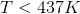The reaction is always spontaneous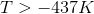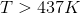Explanation:

A reaction is spontaneous when Gibb's free energy is negative. As a result, we need to determine the temperature range where Gibb's free energy is less than zero. Since we know the values for changes in enthalpy and entropy, we can plug them into the Gibb's free energy equation, and set it equal to zero.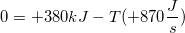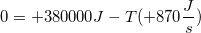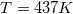437K is the temperature at which Gibb's free energy is zero. Since entropy is positive for this reaction, increasing the temperature will result in a more negative value for Gibb's free energy.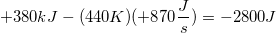As a result, any temperature that is greater than 437K will make this reaction spontaneous.

### Example Question #1 : Laws Of Thermodynamics

Which of the following is a law of thermodynamics?

ΔE (surroundings) = ΔE (system)

The entropy of the universe is always decreasing

ΔS (system) + ΔS (surroundings) = ΔS (universe)

ΔH (system) + ΔH (surroundings) = ΔH (universe)

ΔS (system) + ΔS (surroundings) = ΔS (universe)

Explanation:

The second law of thermodynamics states that the entropy of the system and the entropy of the surroundings is equal to the entropy of the universe. The rest of the answer choices are not one of the fundamental laws of themodynamics.

### Example Question #2 : Laws Of Thermodynamics

Which of the following is true regarding the first law of thermodynamics?

Conversion of kinetic energy to potential energy violates the first law of thermodynamics

It states that the heat lost by a system is gained by the surroundings

It states that the disorder in the universe is always increasing

An exothermic reaction always destroys energy, whereas an endothermic reaction always creates energy

It states that the heat lost by a system is gained by the surroundings

Explanation:

First law of thermodynamics states that the energy of the universe is always constant. This is called the conservation of energy. The total energy of the universe is defined as follows: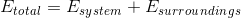According to the law, energy gained or lost by a system is reciprocated by its surroundings. This means that energy, such as heat energy, lost by the system will be gained by the surroundings. This will keep the total energy constant. Energy can be converted from one form to another (such as from kinetic to potential); however, it cannot be created or destroyed.

The second law of thermodynamics states that the disorder of the universe is always increasing. As mentioned, energy can never be created, nor destroyed. In an exothermic reaction, heat energy is transferred from the system to the surroundings. In an endothermic reaction, heat energy is transferred from the surroundings to the system.

### Example Question #3 : Laws Of Thermodynamics

Which of the following substances will have the lowest entropy?

Liquid water

Product of a freezing reaction

Water vapor

Product of a vaporization reaction

Product of a freezing reaction

Explanation:

Entropy is a measure of disorder; therefore, a substance with low entropy will be highly ordered. Gases have the highest entropy because the molecules are spread far apart and are more chaotic. Solids, on the other hand, have the least entropy because they are typically found in an ordered, lattice structure with very little chaos. A freezing reaction involves conversion of a liquid to a solid; therefore, the product of this reaction will have the lowest entropy.

### Example Question #1 : Laws Of Thermodynamics

A researcher creates a perfect crystal and stores it at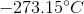. What can you conclude about this substance?

I. The entropy of this substance is less than zero

II. It will have more microstates than gaseous nitrogen

III. Its Gibbs free energy is equal to its enthalpy

I and III

I only

II and III

III only

III only

Explanation:

The third law of thermodynamics states that a perfect crystal at absolute zero (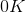or) has an entropy of zero (lowest possible entropy). Note that entropy can never be less than zero.

Microstates are microscopic configurations of a substance, and relates directly to the entropy of the system. The more microstates, the higher the entropy. Since this substance has the lowest entropy it will have the least amount of microstates.

To understand the relationship between Gibbs free energy and enthalpy, we need to look at the following equation:Here,is change in Gibbs free energy,is enthalpy,is change in entropy, and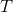is the temperature in Kelvin. From the given information, we know that both temperature and entropy are zero; therefore, Gibbs free energy equals the enthalpy for this substance.

### Example Question #61 : Thermodynamics

A chemistry student is trying to calculate how long it will take a power source of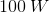to heat a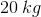sample of ice from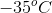to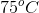. Given that the specific heat capacity of ice is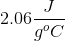, the specific heat capacity of liquid water is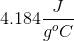, and the heat of fusion for water is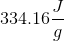, how long will this process take?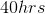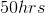There is not enough information to determine the amount of time needed for the process described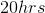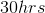Explanation:

In order to solve this problem, we'll need to break it up into steps.

Step 1: Calculate the amount of energy necessary to raise the sample of ice fromto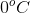. To do this, we'll need to use the following equation: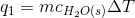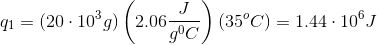Step 2: Calculate the amount of energy necessary to convert the sample atfrom ice to water. We'll need to make use of the following equation: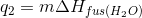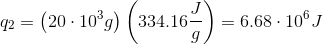Step 3: Calculate the amount of energy necessary to convert the sample of water fromto.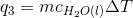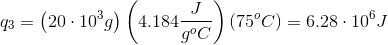Step 4: Sum the amount of energy from the previous 3 steps. This value is the total amount of energy for the entire process.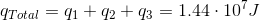Step 5: Now that we know the total amount of energy needed for the process, we need to calculate the time based on the amount of power provided.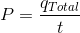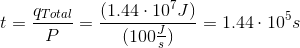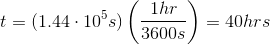### Example Question #1 : Hess' Law

Using the following data to calculate the enthalpy change in:  3 NO2(g) + H2O (l) ->  2 HNO3 (aq) +  NO (g)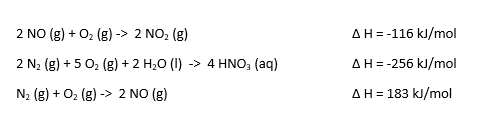-137 kJ/mol

264 kJ/mol

-68.5 kJ/mol

137 kJ/mol

68.5 kJ/mol

-137 kJ/mol

Explanation: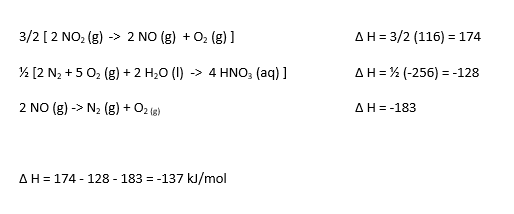### Example Question #2 : Hess' Law

Using the following data to calculate the enthalpy change in:  2 CH4 (g) + 3 O2-> 2 CO (g) + 4 H2O (l)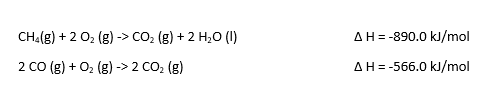-418.4 kJ/mol

-214.2 kJ/mol

-1214 kJ/mol

418.4 kJ/mol

214.2 kJ/mol